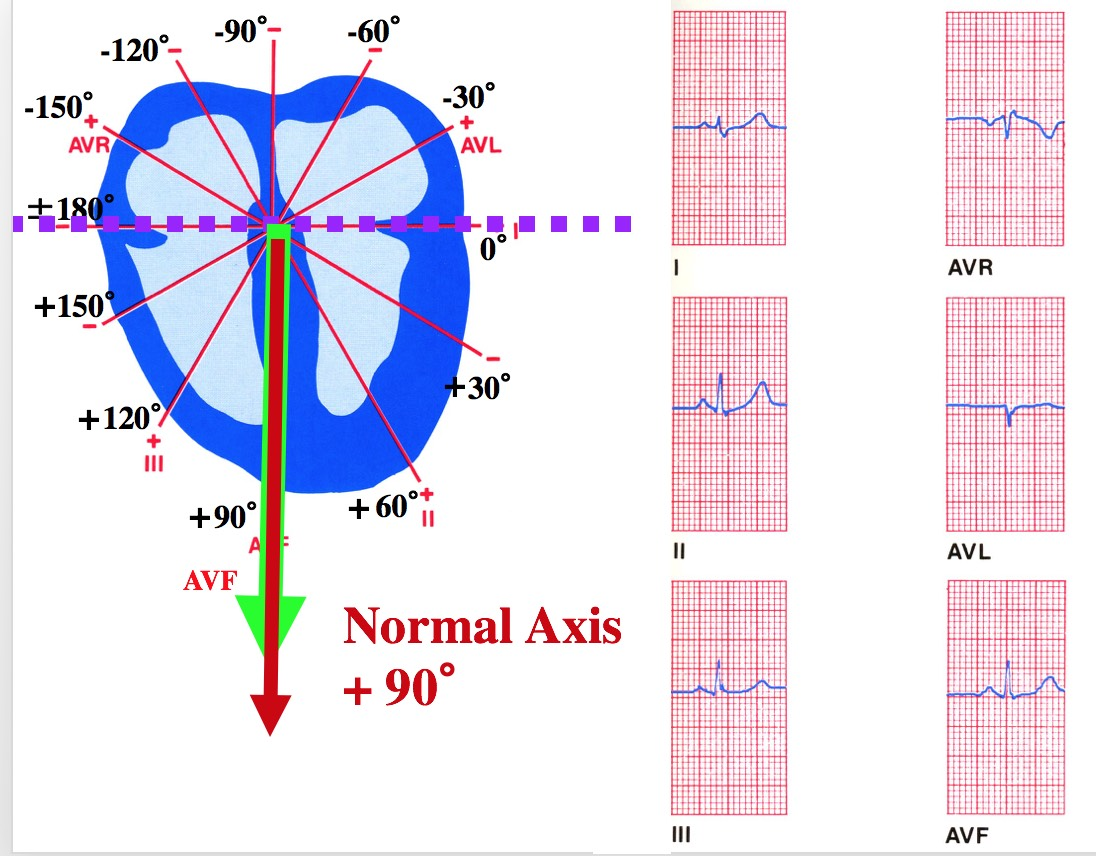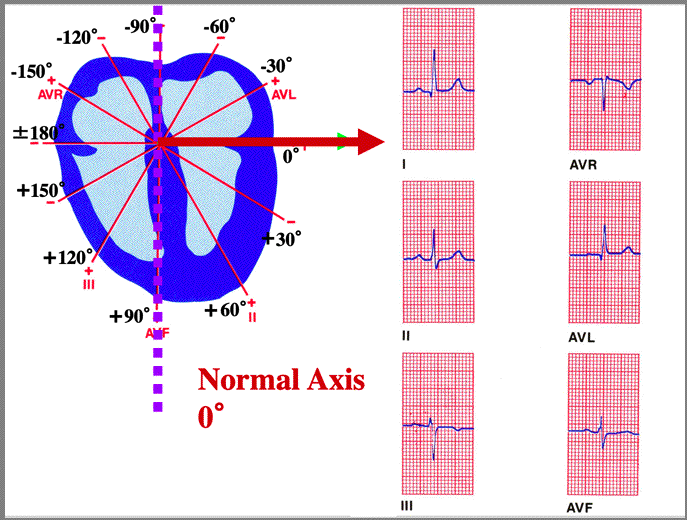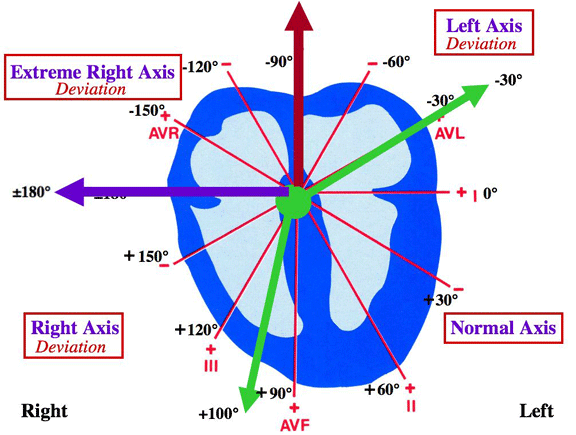EKG PageClass, Below is an example how to determine the QRS axis and degrees when Lead I is the Biphasic (close to isoelectic) LeadSTEP 1: Is the QRS in Lead I mostly positive or negative? LEAD I is the Biphasic Lead (closest to isoelectric)
STEP 2: Is the QRS in AVF mostly positive or negative? POSITIVE
STEP 3: Which lead is the biphasic lead closest to isoelectric. Lead I
REMINDER: THE MEAN QRS AXIS IS ALWAYS PERPENTICULAR TO THE BIPHASIC LEAD. AND, STEP 1 AND STEP 2 HAVE IDENTIFIED THE QUADRANT OF THE MEAN QRS
STEP 4: Determine QRS Axis and degrees? Since Lead I is the biphasic lead and AVF is positive, the classification is Normal Axis +90 DegreesClass, Below is an example how to determine the QRS axis and degrees when AVF is the Biphasic (close to isoelectic) LeadSTEP 1: Is the QRS in Lead I mostly positive or negative? LEAD I is mostly positive
STEP 2: Is the QRS in AVF mostly positive or negative? AVF is the Biphasic lead closest to isoelectric
STEP 3: Which lead is the biphasic lead closest to isoelectric. AVF
REMINDER: THE MEAN QRS AXIS IS ALWAYS PERPENTICULAR TO THE BIPHASIC LEAD. AND, STEP 1 AND STEP 2 HAVE IDENTIFIED THE QUADRANT OF THE MEAN QRS
STEP 4: Determine QRS Axis and degrees? Since AVF is the biphasic lead and Lead I is positive, the classification is Normal Axis 0 Degrees## Review: Mean QRS Axis Classification QuadrantsNormal Axis: from -30 degrees to +100 degrees
Right Axis Deviation: from +100 degrees to ±180 degrees
Extreme Right Axis Deviation: from ±180 degrees to -90 degrees
Left Axis Deviation: from -90 degrees to -30 degreesResearch Interests | Vita | Articles | New Projects | Miscellaneous | UNM | Home